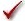## Please enable JavaScript to view this site.

 Navigation: PRINCIPLES OF STATISTICS > Analysis checklists Analysis Checklist: Principal Component Analysis

## Data

###Was the input data free of categorical variables?

PCA will only analyze continuous variables, so categorical variables are simply ignored for data input. If your data table includes categorical variables, they will not be included in the process of generating principal components. However, they can be used for customizing the graphical output (PC Scores plot) of the analysis. This can be helpful for visually identifying specific groups of interest on the PC Scores plot.

## Choices

###If you chose to center (rather than standardize) the data, did you have a good reason?

After standardizing data, each variable (each column) has a mean of 0.0 and a standard deviation (SD) of 1.0. After centering data, each variable has a mean of 0.0, but the SDs won't be the same (each SD will be the same as the SD of the variable being analyzed). Centering alone only makes sense when all of the variables are in the same units and you know that - overall - the variables have the same SD (any differences among SDs are due to random sampling). This is a rare situation, so if you chose centering instead of standardizing, make sure that you can justify this choice. When selecting to center instead of standardize, variables with larger SD will have a greater impact on the analysis, contributing more heavily to the specification of the PCs. However, this may simply be due to measurement scales (inches vs. miles), and thus may ruin the whole point of PCA.

###If you chose a method for selecting components other than parallel analysis, do you have a strong reason?

Statisticians all seem to agree that parallel analysis is a better method for selecting principal components than the other methods that Prism offers. Other, older methods are offered, but these are largely outdated and are only provided as a means to validate previously reported results. Don't use them without a strong reason.

## PCA Results

###How much variation do the selected principal components explain?

We recommend looking at the proportion of variance reported on the tabular results sheet. The goal of PCA is to reduce the number of variables required to describe the data while retaining as much variance from the original data as possible. Look for trends in the proportion of variance explained by the components. If there were correlations (trends) in the original data, the first few components should explain a large proportion of variance, with little variance explained by the last components. If the variables in the original data were nearly or totally uncorrelated (orthogonal), each of the principal components will explain roughly the same amount of variance. In this case, PCA cannot be used to reduce dimensionality, and so likely wasn't needed. This can also be observed using the proportion of variance plot generated by the analysis.